Courses

# Extra Questions- Comparing Quantities Class 8 Notes | EduRev

## Mathematics (Maths) Class 8

Created by: Full Circle

## Class 8 : Extra Questions- Comparing Quantities Class 8 Notes | EduRev

The document Extra Questions- Comparing Quantities Class 8 Notes | EduRev is a part of the Class 8 Course Mathematics (Maths) Class 8.
All you need of Class 8 at this link: Class 8

Question 1: Add: a + b + ab ; b – c + bc and c + a + ac

Solution: We have :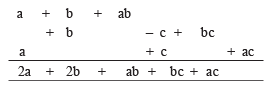Thus, the sum of the given expressions is 2a + 2b + ab + bc + ca.

Question 2: Verify the identity (x + a)( x + b) = x2 + (a + b)x + ab for a = 2, b = 3 and x = 4,

Solution: We have

(x + a)(x + b) = x2 + (a + b)x + ab

Puting x = 2, b = 3 and x = 4, we have

LHS = (x + a)(x + b)

= (4 + 2)(4 + 3)

= 6 * 7 = 42

RHS = x2 + (a + b)x + ab

= (4)2 + (2 + 3)4 + (2 * 3)

= 16 + 5 * 4 + 6

= 16 + 20 + 6 = 42

i.e. LHS = RHS

Thus, the given identity is true for the given values.

Question 3: Using a suitable identity to get the product: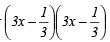Solution: Using the identity (a – b)2 = a2 – 2ab + b2, we have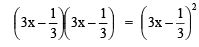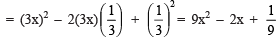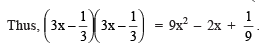Question 4: The length and breadth of a rectangle are 3x2 – 2 and 2x + 5 respectively. Find its area.

Solution: Here,

Length = 3x2 – 2

∴  Area = (Length) * (Breadth)

= (3x2 – 2) * (2x + 5)

= 3x2(2x + 5) + (–2)(2x + 5)

= (3x2 * 2x) + (5 * 3x2) + [(–2) * 2x + (–2) * 5]

= 6x3 + 15x2 + (–4x) + (–10) = 6x3 + 15x2 – 4x – 10

Thus, the required area of the rectangle is 6x3 + 15x2 – 4x – 10 sq. units.

Question 5: Find the volume of cuboid whose dimensions are (x2 – 2); (2x + 2) and (x – 1).

Solution: ∵ Volume of a cuboid = Length * Breadth * Height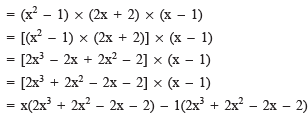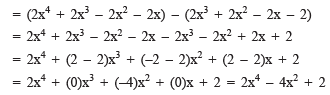,

,

,

,

,

,

,

,

,

,

,

,

,

,

,

,

,

,

,

,

,

;